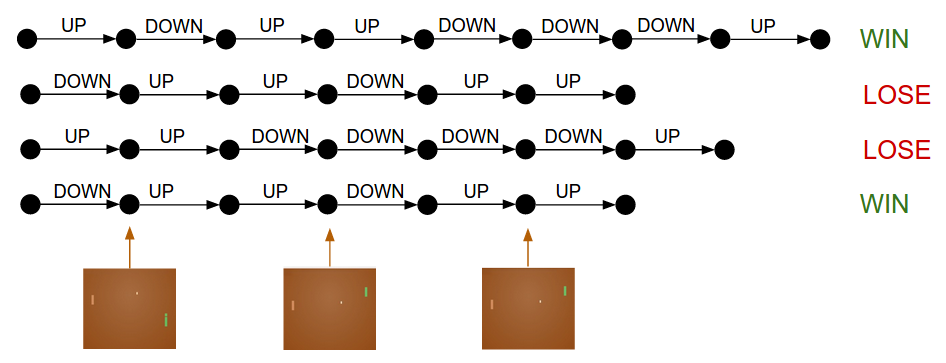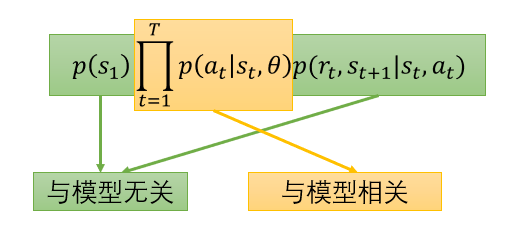## 简介• 球飞来的位置，以及自己球拍的位置等当前游戏环境定义为：state， 用s表示。
• 根据state进行的操作定义为：action, 用a表示。
• 将每次action得到的结果定义为：reward，用r表示。

## Let's Play The Game• 该回合游戏结果 为每一次操作的 reward 加权和(如果简单相加可以将$\gamma$看做1)：
• 将模型根据当前state生成action的函数，也就是我们的 policy 定义$\pi_\theta$为：$p(a_t|s_t, \theta)$• 由于不能枚举所有的情况，所以随机sample出N个回合，对 $R(\theta)$ 进行估计。

## How Can We Play Better?

• $R(\tau^n) > 0$ 时， 为使$R(\theta)$ 加大，调整 $\theta$ 加大 $P(a_t | s_t, \theta)$ ,从而达到效果。
• $R(\tau^n) < 0$ 时， 为使$R(\theta)$ 加大，调整 $\theta$ 减小 $P(a_t | s_t, \theta)$ ,从而达到效果。• 左图，高斯分布的选取点求梯度的图，箭头指向的方向都是函数上升的方向。
• 中图，加入reward之后，会提示选取点哪些方向是不好的，比如红色方向可能会导致函数值下降，所以需要走反方向，而绿色点的方向是函数值上升的方向。
• 右图，经过参数更新后，所有点都向绿色的区域靠近，因为那里可以使函数值上升。